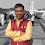### Transistor As Two-port Network

First of all, we have to understand what is a two-port network to understand the transistor as a 2-port network.
Two-port Network:-
Any network with 4 nodes or 4 terminals is considered as a two-port network only if it satisfies the port condition
Port Condition:-
If current entering from one terminal is leaving from some other terminal with the opposite direction but with equal magnitude then these two terminals combine to form a single port. So for a two-port network, we require 4 such terminals that satisfy the port condition.

As you can see above the transistor obeys port condition and therefore we can say that the transistor is like a two-port network.
There are total of 6 parameters for a two-port network but for analysis of the transistor, we will be dealing with only one parameter that is an h-parameter. You can also go with g-parameter
Since,
h=1/g
Now the question arises why we are only using the h-parameter for the analysis of transistors?
So here is the answer to it.
Z-parameter:-
V1 = Z11*I1 + Z12*I2
V2 = Z21*I1 + Z22*I2
By using Z-parameters we can only get to know input and output impedances and we not at all interested in transfer impedances for the analysis of transistors.
Y-parameter:-
I1 = Y11*V1 + Y12*V2
I2 = Y21*V1 + Y22*V2
Similarly, by using Y-parameters we will only get input and output admittance and we not at all interested in transfer admittance
ABCD parameter:-
Vs = A*V + B*I
Is = C*V + D*I
By using ABCD parameters we can just find the source voltage and source current, which is not at all required for the analysis of the transistor.

But now let's talk about h-parameter
V1 = h11*I1 + h12*V2.....(1)
I2 = h21*I1 + h22*V2.....(2)
Now,
From equation (1)
if V2=0 (short-circuited)
h11 = V1 / I1
And as we know voltage by the current is equal to the resistance. Therefore h11 is also known as input impedance (Zi)
if I1=0 (open circuited)
h12 = V1 / V2
So h12 provides reverse voltage gain(1\Av).
So if we took the reciprocal of it then we will get the (Av)voltage gain (V2 / V1) of an amplifier.

Now,
From equation (2)
If V2=0 (short circuited)
h21 = I2 / I1
So h21 parameter provides the current gain of the amplifier.
If I1=0 (open circuited)
h22 =  I2 / V2
So h22 provide output admittance of the amplifier
But if we take the reciprocal of it then we will get (Zo)output impedance (V2 / I2) of the amplifier.

So to study amplifier these 4 parameters plays an important role i.e.
1) Input impedance (Zi).
2) Output impedance (Zo).
3) Voltage gain (Av). &
4) Current gain (Ai).

Just see the video given below it will give you a great idea about the parameters to be used while designing any amplifier.

So in ac analysis or for small-signal analysis, the transistor model can be drawn as shown below.

hie:-represents input impedance of transistor.
hre:-represents reverse voltage gain of the transistor.
hfe:-represents forward current gain of transistor it is also known as (b).
hoe:-represents open circuit or admittance of transistor.
(e) which is used in the above parameters represents that we are doing ac analysis of common emitter configuration.
Now,
V1=Vin of the transistor which is in few millivolts while V2=Vce or output voltage which might be in volts but greater than Vin
Therefore h12=hre=V1/V2 is almost equals to zero (h12=hre=0).Thus it can be neglected.
Also,
I2=Ic or the output current of the transistor is in a few milli-amperes while the V2=Vce or output voltage which might be in volts
therefore h22=hoe=I2/I1 is almost equals to zero (h22=hoe=0).
So 1/hoe becomes very large. Thus it can be replaced by an open circuit.
So the approximate model of the BJT transistor can be drawn as shown below.
I hope you understood it. If you like it then please let me know in the comment section.

1.1.